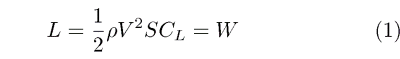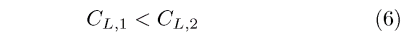## FLIES FASTER

Ever wonder why a sailplane with low drag "flies faster" than a sailpane with high drag? Probably not, since it makes perfect sense. But does it? From the well-known equation for the liftwe can writeThis equation shows that the speed depends on the wing loading (W/S), density, and lift coefficient. The speed does not depend on the drag – a result that does not seem to make the perfect sense we expected. The equation is correct, but the approach is incorrect.

The flaw is related to what is meant by "flies faster." When we directly compare two sailplanes in flight side-by-side, we fly them at approximately the same glide slope (or L/D). In other words, we do not match the lift coefficient CL but instead the L/D, which equals the ratio CL/CD. For the two aircraft, this requirement giveswhere the subscript (1) refers to the sailplane with the lower drag and (2) the one with the higher drag. Equation (3) gives:Sincewe get the result thatThus, if we assume that the two sailplanes have the same wing loading, then through equation (1) we find that the speed V of the sailplane with the lower drag (flying at CL1) is higher than that of the sailplane with the higher drag (CL2) – a result that agrees with the "right answer" – our expectation.

RC Soaring Digest Homepage